# Python | Pandas Series.where

Python is a great language for doing data analysis, primarily because of the fantastic ecosystem of data-centric python packages. Pandas is one of those packages and makes importing and analyzing data much easier.

Pandas series is a One-dimensional ndarray with axis labels. The labels need not be unique but must be a hashable type. The object supports both integer- and label-based indexing and provides a host of methods for performing operations involving the index.

Pandas` Series.where()` function replace values where the input condition is `False` for the given Series object. It takes another object as an input which will be used to replace the value from the original object.

Syntax: Series.where(cond, other=nan, inplace=False, axis=None, level=None, errors=’raise’, try_cast=False, raise_on_error=None)

Parameters :
cond : boolean NDFrame, array-like, or callable
other : scalar, NDFrame, or callable
inplace : boolean, default False
axis : int, default None
level : int, default None
errors : str, {‘raise’, ‘ignore’}, default raise
try_cast : boolean, default False

Returns : wh : same type as caller

Example #1: Use `Series.where()` function to replace values in the given Series object with some other value when the passed condition is not satisfied.

 `# importing pandas as pd ` `import` `pandas as pd ` ` `  `# Creating the First Series ` `sr1 ``=` `pd.Series([``'New York'``, ``'Chicago'``, ``'Toronto'``, ``'Lisbon'``, ``'Rio'``]) ` ` `  `# Creating the row axis labels ` `sr1.index ``=` `[``'City 1'``, ``'City 2'``, ``'City 3'``, ``'City 4'``, ``'City 5'``]  ` ` `  `# Print the series ` `print``(sr1) ` ` `  `# Creating the second Series ` `sr2 ``=` `pd.Series([``'New York'``, ``'Bangkok'``, ``'London'``, ``'Lisbon'``, ``'Brisbane'``]) ` ` `  `# Creating the row axis labels ` `sr2.index ``=` `[``'City 1'``, ``'City 2'``, ``'City 3'``, ``'City 4'``, ``'City 5'``] ` ` `  `# Print the series ` `print``(sr2) `

Output :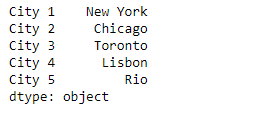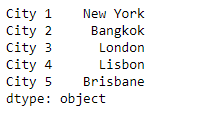Now we will use `Series.where()` function to replace those values which does not satisfy the passed condition.

 `# replace the values ` `sr1.where(sr1 ``=``=` `'Rio'``, sr2) `

Output :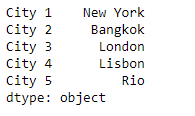As we can see in the output, the `Series.where()` function has replaced the names of all cities except the ‘Rio’ city.

Example #2 : Use `Series.where()` function to replace values in the given Series object with some other value when the passed condition is not satisfied.

 `# importing pandas as pd ` `import` `pandas as pd ` ` `  `# Creating the First Series ` `sr1 ``=` `pd.Series([``22``, ``18``, ``19``, ``20``, ``21``]) ` ` `  `# Creating the row axis labels ` `sr1.index ``=` `[``'Student 1'``, ``'Student 2'``, ``'Student 3'``, ``'Student 4'``, ``'Student 5'``] ` ` `  `# Print the series ` `print``(sr1) ` ` `  `# Creating the second Series ` `sr2 ``=` `pd.Series([``19``, ``16``, ``22``, ``20``, ``18``]) ` ` `  `# Creating the row axis labels ` `sr2.index ``=` `[``'Student 1'``, ``'Student 2'``, ``'Student 3'``, ``'Student 4'``, ``'Student 5'``] ` ` `  `# Print the series ` `print``(sr2) `

Output :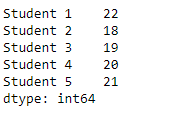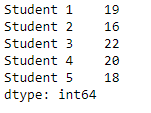Now we will use `Series.where()` function to replace those values which does not satisfy the passed condition.

 `# replace the values ` `sr1.where(sr1 >``20``, sr2) `

Output :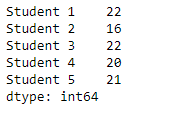As we can see in the output, the `Series.where()` function has replaced all the values which did not satisfy the passed condition.

My Personal Notes arrow_drop_upCheck out this Author's contributed articles.

If you like GeeksforGeeks and would like to contribute, you can also write an article using contribute.geeksforgeeks.org or mail your article to contribute@geeksforgeeks.org. See your article appearing on the GeeksforGeeks main page and help other Geeks.

Please Improve this article if you find anything incorrect by clicking on the "Improve Article" button below.

Article Tags :

Be the First to upvote.

Please write to us at contribute@geeksforgeeks.org to report any issue with the above content.